Faqs | Help | @ | Contact |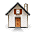## Robust decision making in the face of severe uncertainty

My interest in robust decision-making centers primarily on the modeling of decision-making problems that are subject to conditions of severe uncertainty. The discussion here is related to my Info-Gap campaign.

## Overview

The term Robustness seems to have an immediately familiar ring to it. Still, to be able to use it meaningfully in a discussion on robust decision-making it is necessary to be clear on the purport one ascribes it in the discussion. Consider then this definition from WIKIPEDIA:
Robustness is the quality of being able to withstand stresses, pressures, or changes in procedure or circumstance. A system, organism or design may be said to be "robust" if it is capable of coping well with variations (sometimes unpredictable variations) in its operating environment with minimal damage, alteration or loss of functionality.

Clearly, the main drift of this definition is that robustness signifies "resilience to change/variation/pressure". The question is then how would we define this idea formally? Furthermore, how exactly would we define a "robust" solution to a problem, say a decision-making problem?

Surprisingly, the discussion on these issues in the relevant literatures is rather scant. The prevailing tendency there is to rush to the "solution" of the problem considered, thus indicating that this is where the "real" action is, or ought to be. Contrary to this practice, my aim here is to discuss some aspects of the modeling of robust decision-making with the view to ultimately incorporate this material in my planned book on ... robust decision-making.

I wish to stress that my treatment of the topic here is formal. I strongly believe that the need for such a treatment is real and timely because all too often the term "robust" is used as no more than a buzzword with a web of empty rhetoric spun around it.

Also, the term "robust" can mean different things to different people. Furthermore, its exact meaning is very much context dependent. It is important, therefore, to explain/define what this term means and how it should be interpreted in the context of the decision-making situation under consideration.

It is heartening therefore to see that this message comes through loud and clear in this assessment given by an "official body":

... Another concern of the committee regarding the content of this chapter involves the use of the concept of "robustness." The committee finds that this term is insufficiently defined. A plausible argument can be made that there is no meaningful distinction from usual optimality analysis and that the concept discussed in this report is a matter of a poorly defined utility function. If indeed there is a real technical distinction to be made, the authors should consider expanding and supporting the discussion of this concept. Furthermore, the committee suggests that the authors address the concept of adaptive management in conjunction with discussions of robustness and in particular address how different sources of uncertainty affect different kinds of decisions. Finally, the committee would appreciate a further elucidation of what the author considers to constitute "deep uncertainty" (page 34 and other locations). The committee understands that there is overlap between this concept and the others defined in this section (e.g., "robust"), but nevertheless finds that it is not entirely clear when the author considers the situation inappropriate for use of conventional methods for characterizing uncertainty.

Review of the U.S. Climate Change Science Program's Synthesis and Assessment Product 5.2
"Best Practice Approaches for Characterizing, Communicating, and Incorporating
Scientific Uncertainty in Climate Decision Making"
pp. 17-18, 2007
http://www.nap.edu/catalog/11873.html

In short, ..., if you are here for buzzwords, then you are in the wrong place, mate!

So, as indicated above, I understand robust decision-making to be concerned with problems whose object is to identify decisions that are resilient to changes in the "environment" in which they are made. These changes can be due to uncertainty and/or (controlled) variability.

## Example

Consider the following two shortest path problems, where in both cases the objective is to determine a path from node 1 to node 4.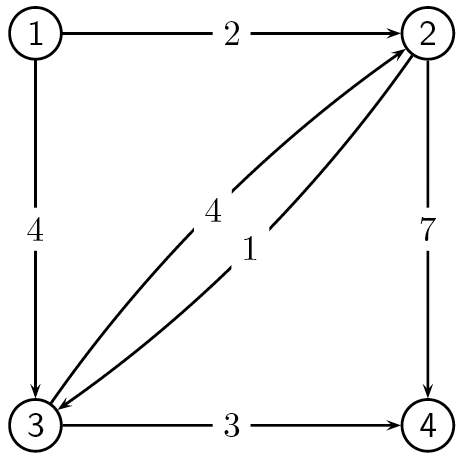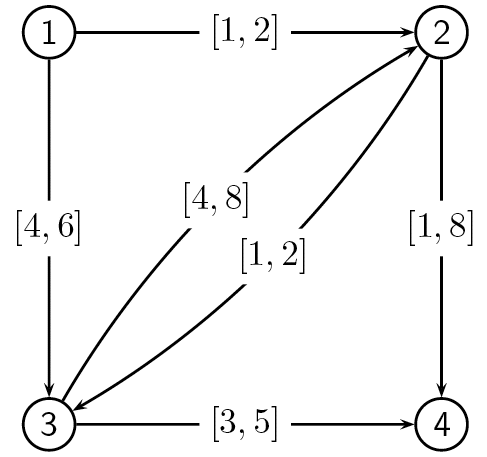This is the conventional version of the problem. We ask: what is the shortest path from node 1 to node 4? Note that the length of each arc is completely specified. This is the "robust-counterpart" of the conventional problem. We ask: what is the shortest robust path from node 1 to node 4? Note that here the length of an arc is specified by an interval rather than a single number. This interval stipulates the range of values that the length of the arc can take. It thus represents the uncertainty/variability in the parameter of interest.

## The mighty Maximin

In this discussion the "context" is a formal decision problem, which can be either a satisficing problem and/or an optimization problem. So, mathematically speaking, we shall consider robustness with repect to an objective function and/or constraints.

To account for the fact that changes with consequences for robustness may be reflected differently in the constraints than in the objective function, it is instructive to distinguish between

• Robust-satisficing

• Robust-optimizing

The former refers to robustness with respect to constraints, whereas the latter refers to robustness with respect to the objective function of an optimization problem.

The key technical term here is "for all" and the key symbol is "V" . For example, the following is a simple (abstract) robust-satisficing problem:

Find an x' in X such that g(x',u) >= 0 for all u in U.

Here X represents the set of decisions available to us, U represents the set of values that a parameter u can take and g is a real-valued function on X x U. In words, we have to find a decision x' in X that is robust with respect to the constraint g(x',u) >= 0 in that x' should satisfy this constraint for all u in U.

And here is a simple (abstract) robust-optimizing problem:In words, the problem is to find a solution x in X whose worst-case value of f(x,u) over u in U is the largest possible. So, another key term in this area is the concept "worst case".

When it comes to framing such decision problems in terms of a mathematical model, Wald's (1945, 1950) famous Maximin model and its many offspring still dominate the scene. The above robust-optimization model is one of the formats of Wald's model. The most familiar format of this model is as follows: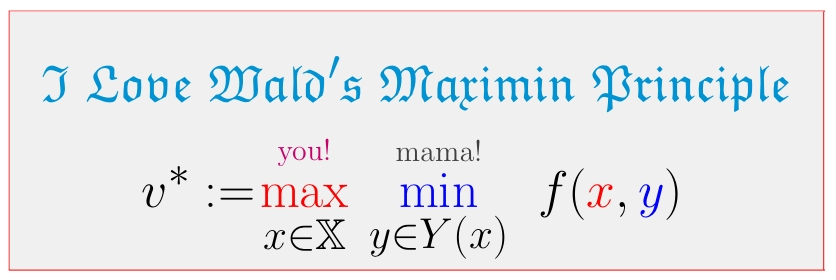In this framework, the outer player represents the decision maker (you!) and the inner player represents Nature (uncertainty). The outer player plays first, and based on her decision (x) the inner player selects her decision (y).

Interestingly, some purportedly new robustness models — such as the one proposed by Info-Gap decision theory — are Maximin models par excellence.

Some of the modeling issues encountered in robust decision-making are discussed in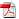Anatomy of a Misguided Maximin formulation of Info-Gap's Robustness ModelI plan to post on this page results of my research in this area. Since I am doing research in other areas as well, updates of this page may not be all that frequent.

## Classification of robustness

I use two different criteria to classify robust decision-making problems, namely

Object of interest Type of Coverage Objective functionConstraints CompletePartialLocal

The object criterion distinguishes between robustness with respect to constraints and robustness with respect to the objective function of an optimization problem. The coverage criterion distinguishes between three types of robustness in relation to the region of variability/uncertainty over which robustness is sought.

This simple classification induces the following classes of robustness problems:

• Object:
• Robust-optimizing problems
• Robust-satisficing problems
• Robust-optimizing-satisficing problems

• Coverage:
• Complete robustness problems
• Partial robustness problems
• Local robustness problems

As far as terminology is concerned, I use a (coverage,object) pair to denote the type of robustness under consideration. So here is the list of the nine types of robustness problems induced by the above classification scheme:

• Complete robust-optimizing problems
• Complete robust-satisficing problems
• Complete robust-optimizing-satisficing problems
• Partial robust-optimizing problems
• Partial robust-satisficing problems
• Partial robust-optimizing-satisficing problems
• Local robust-optimizing problems
• Local robust-satisficing problems
• Local robust-optimizing-satisficing problems

More details on this classification can be found in the articleTo explain why the need for the "coverage" criterion arises at all, consider the following robust-satisficing problem, where X and U are some given sets and g is a real-valued function on X x U:

Find an x' in X such that g(x',u) >= 0 for all u in U

The point is that the requirement "for all u in U" is often too demanding. In other words, if we insist on "complete robustness" against variability in the value of u with respect to the constraint g(x',u) >= 0, then the problem might not have a feasible solution: there might be no such x' in X.

In such cases we may have to make do with less demanding requirements and settle for a "partial" robustness:

Find an x' in X such that g(x',u) >= 0 for all u in a large subset of U

And there are cases where we are interested in the variability in u only in the immediate neighborhood of some given element u* of U. In this case the robustness will be "local" in nature:

Find an x' in X such that g(x',u) >= 0 for all u in the immediate neighborhood of u*

Note that in the "partial" case we have to specify/quantify what we mean by "large subset of U" and in the case of "local" robustness we have to specify/quantify what we mean by "immediate neighborhood of u*". These are very important modeling issues that I shall address in due course.

For now I note that it is immediately clear that one obvious approach is to require, in these cases, the "largest possible robustness". So in the case of "partial" robustness we can require the "large subset" to be the "largest possible subset" (The origin of this approach dates to Starr's (1962, 1966) "domain criterion". See discussion on this and related models in my The Mighty Maximin! paper).

The recipe is then as follows:

### Size Criterion

Let
A(x):={u in U: g(x',u) >= 0}, x in X,
and define the robustness of decision x as the "size" of A(x), according to a suitable measure of "size". The larger the "size" of A(x) is, the better (more robust) x is, hence the most robust decision is one whose A(x) set is the largest.

Formally then, we can define the robustness of decision x as follows:

 ρ(x):= max {size(V): g(x,u)>=0, for all u in V } V⊆U

where size(V) denotes the size of set V.

Similarly, in the case of "local" robustness we can require the "immediate neighborhood" to be the "largest immediate neighborhood".

Needless to say, "local" robustness is a special case of "partial" robustness, and in some cases the distinction between them is blurred.

So, you be the judge as to which of the following coverages is partial and which is local. For your convenience, the mathematical expressions (constraints) generating these beauties are also provided. In all cases, the complete region of uncertainty (U) is the rectangle specified by the vertices (-1.5,-1.5),(2,-1.5),(2,2),(-1.5,2).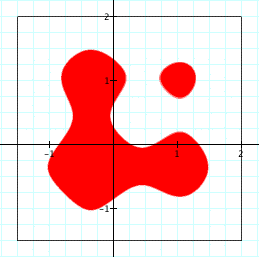x2 + y2 + sin(4x) + sin(4y) <= 1x + cos(3x) - cos(3y) <= 0(x-0.5)2 + (y-0.5)2 <= 1Do it on your own

And while you are at it, determine which coverage is the most robust.

At this stage I should note how the uncertainty comes into the picture. The uncertainty pertains to the set U which represents the range of values that a parameter of the model concerned can take. Thus, what is known is that the "true" value of this parameter is in set U. The big unknown is: which element of U is the "true" value of the parameter.

If the uncertainty is very mild then we may have a good estimate of the true value of u, and therefore may seek to restrict the robustness analysis to the immediate neighborhood of this estimate.

In contrast, if the uncertainty is severe, then the estimate we may have is expected to be a wild guess, a poor indication of the true value of u, and is likely to be substantially wrong. For this reason, as I have been arguing all along, a "local" robustness analysis in the immediate neighborhood of this poor estimate cannot possibly count as a proper treatment of severe uncertainty. In fact, such an approach amounts to voodoo decision-making.

As we know from the distinction drawn in optimization theory between local and global analyses, a "local" analysis cannot provide an assessment of the overall situation. By the same token, in the case of robustness, a local robustness analysis can yield the robustness of an object, state-of-affairs etc. only with respect to the locale in which the analysis is conducted. But this may give a very distorted assessment of the robustness of the object etc with regard to the complete region of uncertainty under consideration.

The picture speaks for itself and requires no elaboration.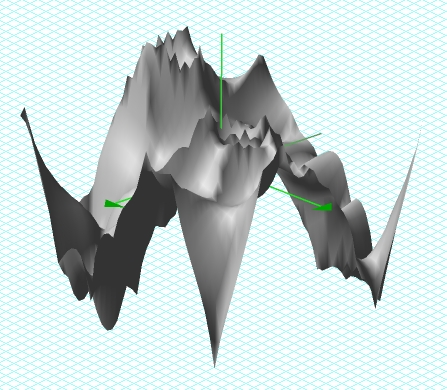You cannot obtain a good picture of Australia's robustness from an analysis of the resilience of Melbourne and its immediate vicinity.

The following quote alludes to the distinction between local and nonlocal robustness and the manner in which they should be modeled:

2. Basic theory

In this section, we describe a general framework for local robustness analysis. By local robustness analysis, we refer to the idea that a policymaker may not know the 'true' model for some outcome of interest, but may have sufficient information to identify a space of potential models that is local to an initial baseline model. This may be regarded as a conservative approach to introducing model uncertainty into policy analysis, in that we start with a standard problem (identification of an optimal policy given a particular economic model) and extend the analysis to a local space of models, one that is defined by proximity to this initial baseline. The local model uncertainty assumption, in our judgment, is naturally associated with minimax approaches to policy evaluation. When a model space includes nonlocal alternatives, we would argue that one needs to account for posterior model probabilities in order to avoid implausible models from determining policy choice.

W.A. Brock, S.N. Durlauf (page 2070)
Local robustness analysis: Theory and application
Journal of Economic Dynamics & Control 29, 2067–2092, 2005.

It also identifies the major difficulty in modeling global robustness, namely the treatment of rare events with high impact (Black Swans).

So, as one might expect, the robustness models offered by classical decision theory and robust optimization for the treatment of severe uncertainty, take full account of this crucial concern to the effect that the robustness analyses that they set out are obviously non-local. It is also not surprising that these models are often not "purely" minimax/maximin models.

## Info-Gap Decision Theory

A striking exception to the above rule is Info-Gap's robustness model. The distinguishing characteristic of this model is that it is utterly oblivious to the considerations that must be taken into account in a robustness analysis in the face of severe uncertainty. Thus, the robustness analysis that it prescribes amounts to no more than fixing on a single estimate, that (subject as it is to severe uncertainty) is a "wild guess", and on the neighborhood of this "wild guess".

As the results of such an analysis cannot be better than "wild guesses", the inevitable conclusion is then that Info-Gap's robustness model is thoroughly unsuitable for decision-making under severe uncertainty. The following example illustrates this point.

Example:Suppose that you want to select a decision q from a set Q to satisfy the performance requirement R(q,u) >= 0, where R is a real- valued function that depends on q but also on some parameter u.

Now suppose that the true (correct) value of u is subject to severe uncertainty. In fact, suppose that all we know about the true value of u is that it is ... a real number.

Next, consider two decisions, q' and q'', and assume that

• R(q',u) = 1+6u2-|u|

• R(q'',u) = 0.2 - 4u2

So the question is: which of the two decisions is more robust with respect to the performance requirement R(q,u)>= 0 ?

Since the uncertainty regarding the true value of u is severe, we argue as follows:

• q' is very robust.
Indeed, it satisfies the performance requirement essentially over the entire given region of uncertainty U:= (-infinity,infinity), except for two very small subintervals located in [-1,1].
Furthermore, R(q',u) > R(q'',u) over most of the given region of uncertainty U.
In short, q' seems to be a clear winner here: it is by far more robust than q'' with respect to the constraint R(q,u) >=0 over the region of uncertainty under consideration.

• q'' is very fragile.
indeed, q'' violates the performance requirement essentially over the entire given region of uncertainty except for a very small interval around the origin.

The verdict seems clear: q' is the undoubted winner.

Now suppose that we turn to Info-Gap decision theory to answer this question.

Then the first thing we have to do is to determine an estimate for the true value of u. Of course, under severe uncertainty it might be impossible to compute a reliable estimate for the true value of u. For as we pointed out above, these are the properties of such an estimate:

• A wild guess.

• Poor indication of the true value of u.

• Likely to be substantially wrong.

But, no worries. This seems to be fine by Info-Gap decision theory.

So suppose that in our case, we argue by symmetry that the best estimate of the true value of u is u = 0. In this case Info-Gap's robustness model will select q'' as the most robust decision, yes — q''.

Why?

Because according to Info-Gap decision theory, the robustness of a decision is equal to the size of the largest region of uncertainty, in the neighborhood of the estimate, over which the performance constraint is satisfied.

On the face of it, following Info-Gap's requirements, the robustness of a decision would depend on how Info-Gap's nested regions of uncertainty are set up. But this will not change the preference: q'' is slightly more robust than q'. This is so because as indicated by the graph R(q',u) becomes negative closer to the estimate . This can be seen clearly if we zoom in on the estimate: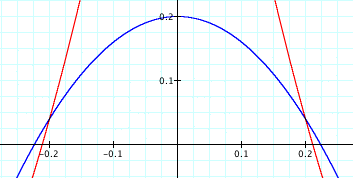Example:

This example illustrates the difference between partial and local robustness. It was constructed by my student, Daphne Do, for her honors thesis in 2008.

Consider a situation where two decisions, call them x and y have — according to info-gap's robustness model — the same robustness in the neighborhood of the estimate. The following picture shows how local robustness is determined by an info-gap robustness model.As you can no doubt see, relative to the total region of uncertainty, decision y is far more robust than decision x. In other words, the partial robustness of y is much larger than the partial robustness of x.

However, locally around the estimate, these two decisions have the same robustness (radius of the circle around the estimate).

The implication is then that Info-Gap's robustness model does not even begin to deal with the severity of the uncertainty under consideration. All it prescribes is to examine the area around the estimate and to call it a day!

In the language of the Land of No Worries,

 Decision-Making Under Severe Uncertainty a la Info-Gap Decision Theory Fundamental Difficulty Robust Solution The estimate we have is a wild guess, a poor indication of the true value of the parameter of interest, and is likely to be substantially wrong. No worries, mate! Conduct the analysis in the immediate neighborhood of the estimate!

One wonders then how such a prescription for robustness against severe uncertainty can be contemplated at all.

In short, isn't it clear that this prescription is totally oblivious to the limitations of a local analysis? Furthermore, that it openly violates the Garbage In — Garbage Out Axiom. This is the reason for my dubbing Info-Gap decision theory a Voodoo Decision Theory par excellence.

And to top it all off, not only do Info-Gap scholars ignore (or, fail to see) these obvious flaws, those who should know better, continue to promote this theory from the pages of professional journals. See my discussion on Info-Gap Economics.

As many readers would no doubt know, the concept "Stability Radius" is a well-known, and well established idea. It is used in a variety of fields to designate local stability/robustness. You may wonder, therefore, why this concept is discussed in some detail on a page that is dedicated to robustness against severe uncertainty.

The reason for this is simple. I take up this concept in this context to point out (to the unwary reader of the Info-Gap literature) the following fact.

What in the Info-Gap literature is referred to as "Info-Gap's robustness", is known to “the rest of the world” as stability radius. That is, Info-Gap's measure of robustness is nothing more and nothing less than the stability radius of the feasible region defined by Info-Gap's performance requirement. In other words, according to Info-Gap decision theory, the robustness of a decision is effectively equal to the stability radius of the "safe region" associated with the decision.

My objective in calling attention to this fact is not only to point out that Info-Gap’s central concept, namely its measure of robustness, is in fact the exact equivalent of a well-known, already well-established, concept. But to bring out more forcefully, by way of comparison, the absurd in the Info-Gap approach to the treatment of severe uncertainty. The point is this:

The concept "radius of stability" designates a means for determining local stability. The idea here is that the stability measured here is obtained against small perturbations from a given nominal value. This means of course that nowhere in the fields where it is used is this concept invoked to measure global stability.

An exception to this rule is Info-Gap. Info-Gap has the dubious distinction of being the only method that invokes the “radius of stability" to determine global robustness. That is, in Info-Gap this measure of robusntess is invoked to evaluate the robustness of decisions against severe uncertainty. The reason that this comparison between Info-gap's approach and that of “the rest of the world” highlights the absurd underlying Info-Gap decision theory is obvious. Info-Gap's prescription to use a local Robustness measure as a means for evaluating robustness against severe uncertainty flies in the face of the very nature of decision-making under severe uncertainty. Conditions of severe uncertainty demand that robustness be sought to be determined globally. But, by prescribing that robustness against severe uncertainty be governed by a local measure of Robustness, Info-gap's prescription constitutes the exact antithesis of what such a prescription ought to be. As there is no mention whatsoever of the concept stability radius in the official Info-Gap literature, it is important to discuss this concept, however briefly, in this context.

So, here is a very abstract, non-technical description of this important notion.

Consider a system that can be in either one of two states: a stable state or an unstable state, depending on the value of some parameter p. We also say that p is stable if the state associated with it is stable and that p is unstable if the state associated with it is unstable. Let P denote the set of all possible values of p, and let the "stable/unstable" partition of P be:

• S = set of stable values of p. We call it the region of stability of P.

• I = set of unstable value of p. We call it the region of instability of P.

Now, assume that our objective is to determine the stability of the system with respect to small perturbations in a given nominal value of p, call it p'. In this case, the question that we would ask ourselves would be as follows::

How far can we move away from the nominal point p' (under the worst-case scenario) without leaving the region of stability S?

The "worst-case scenario" clause determines the "direction" of the perturbations in the value of p': we move away from p' in the worst direction. Note that the worst direction depends on the distance from p'. The following picture illustrates the simple concept behind this fundamental question.Consider the largest circle centered at p' in this picture. Since some points in the circle are unstable, and since under the worst-case scenario the deviation proceeds from p' to points on the boundary of the circle, it follows that, at some point, the deviation will exit the region of stability. This means then that the largest "safe" deviation from p' under the worst-case scenario is equal to the radius of the circle centered at p' that is nearest to the boundary of the region of stability. And this is equivalent to saying that, under the worst-case scenario, any circle that is contained in the region of stability S is "safe".

So generalizing this idea from "circles" to high-dimensional "balls", we obtain:

The radius of stability of the system represented by (P,S,I) with respect to the nominal value p' is the radius of the largest "ball" centered at p' that is contained in the stability region S.

The following picture illustrates the clear equivalence of "Info-Gap robustness" and "stability radius":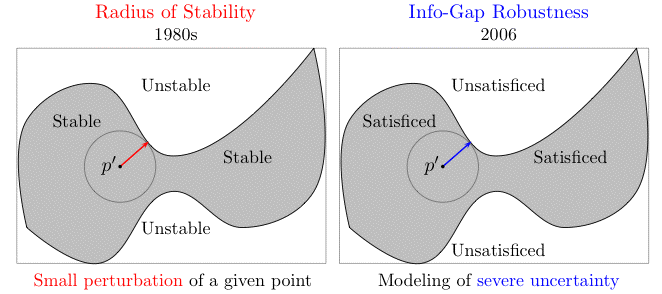In short, for all the spin and rhetoric, hailing Info-Gap's local measure of robustness as new and radically different, the fact of the matter is that this measure is none other than the "old warhorse" known universally as stability radius.

And as pointed out above, what is lamentable about this state-of-affairs is not only the fact that Info-Gap scholars fail to see (or ignore) this equivalence, but also that those who should know better, continue to promote this theory from the pages of professional journals. See my discussion on Info-Gap Economics.

This topic is related to some of my other campaigns, namely the Worst-Case Analysis / Maximin Campaign and the Info-Gap Campaign.

More on this and related topics can be found in the pages of the Worst-Case Analysis / Maximin Campaign, Severe Uncertainty, and the Info-Gap Campaign.

## Recent Articles, Working Papers, Notes

Also, see my complete list of articles

## Recent Lectures, Seminars, Presentations

If your organization is promoting Info-Gap, I suggest that you invite me for a seminar at your place. I promise to deliver a lively, informative, entertaining and convincing presentation explaining why it is not a good idea to use — let alone promote — Info-Gap as a decision-making tool.

Here is a list of relevant lectures/seminars on this topic that I gave in the last two years.

Disclaimer: This page, its contents and style, are the responsibility of the author (Moshe Sniedovich) and do not represent the views, policies or opinions of the organizations he is associated/affiliated with.